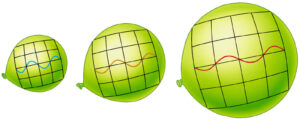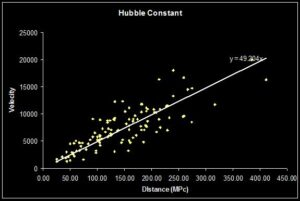# The Universe Expands Far Faster Than Anticipated…

##The Universe is accelerating

For a while now, astrophysicists have known that our Universe is expanding, and accelerating.  And much like the surface of a rubber balloon getting inflated, space is getting bigger, and bigger, and bigger…

Two experiments have shown a large discrepancy between theory and observation.  The new astronomical measurements obtained by NASA and the European Space Agency (ESA) suggest that the Universe is in fact expanding quicker than the laws of Physics should allow.

Scientists have established that this discrepancy is not the result of an accident during the measurement process, but rather a fact of our unstable Universe – which is expanding 9% faster than theory predicts it should according to theoretical computer models.

## Intergalactic Space is ExpandingThe Balloon analogy illustrates what happens to the wavelength of light when the Universe expands.  As the wavelength of light increases, a red-shift is observed (right).  As the wavelength of light decreases, a blue-shift is observed (left).

Like dots pencilled on the surface of a party balloon, space between galaxies itself is stretching.

Imagine that you are an object on the surface of a balloon and everything is moving away from you because the balloon is getting bigger.  That’s all well and good, but the balloon itself is expanding into a dimension that one, on the surface, does not directly experience.

Nevertheless, astronomers can observe this cosmological expansion by measuring the light from distant galaxies and supernovae, which turns redder as it passes through this stretching space!

## A Shift in Colour

By measuring this “redshift“, scientists can calculate the rate of expansion – but astronomers find a baffling discrepancy between what science predicts and what it actually observes.

### Red-Shift, Blue-Shift

The ‘Equation of Light‘ is$\lambda = \frac{c}{\nu}$

where$\lambda$ is the wavelength of the light.And so we can see that…

Wavelength is inversely proportional to frequency.

#### Red: Away from the Observer

A shift in colour to lower frequencies, or longer wavelengths (the red end of the spectrum) is an indication of motion away from the observer.

#### Blue: Towards the ObserverThe Doppler effect stretches the light wave towards the red end of the spectrum, or compresses the wave towards the blue end of the spectrum.

A shift in colour to higher frequencies, or shorter wavelengths (the blue end of the spectrum) is instead an indication of motion towards the observer.

### The RedShift z

To measure the shift in wavelength and determine the redshift z, we compare the change in wavelength$\Delta \lambda$ to the original wavelength or ‘rest’ wavelength$\lambda$ of a galaxy.

So that,$z = \frac{\Delta \lambda}{\lambda_0}$

It follows that…

If the wavelength of a line in the light spectrum of a galaxy or a supernova is measured by observation, then compared with the wavelength of the same spectral line, as measured in a laboratory, the red-shift or blue-shift of the galaxy can be calculated.Note The redshift/blueshift is related to the speed of motion$v$ of the observed astrophysical object.  It can also be converted into the speed of recession or approach of that galaxy.$z = \frac{v}{c}$

## Reviewing the Cosmological Constant

### Hubble Constant and CMBThe Hubble constant H0 is given by the speed of recession v over r the distance to the receding galaxy (km s-1 Mpc-1).

The Hubble constant H0 is the proportionality factor between the distance of a galaxy and the speed it moves away from us.

Galaxies that are further away move away increasingly faster, as expected from the expansion of space.

Without going into hair-rising algebraic details, it means that for every Megaparsec (Mpc) further away you look, galaxies appear to speed away faster by H0 kilometres per second.$H_0 = \frac{v}{r}$

Now H0 can be measured in two ways using:

• the Cosmic Microwave Background (CMB) or
• the cosmic distance ladder.

The most recent measurement made by the Planck satellite mission was published back in July 2018, when the final results gave a value of

H0 = 67.66 ± 0.42 km s−1Mpc−1.

However, astronomers who measure the speed and distance (with the ‘standard candles’ method) of galaxies think the value is closer to

H0 = 73.52 ± 1.62 km s−1 Mpc−1.

## Beyond a Plausible Level of Chance

### The Hubble Tension

The Hubble tension between the early and late universe may well be the most exciting development in cosmology for decades.

That is the opinion of Adam Riess, the lead researcher, of a new scientific paper on ‘The Local Perspective on the Hubble Tension: Local Structure Does Not Impact Measurement of the Hubble Constant‘, published in The Astrophysical Journal.

In 2011, Professor Adam G. Riess and his colleagues won the Nobel Prize in Physics for providing evidence that the expansion of the universe was accelerating.

## A Baffling Astronomical Discrepancy

The new value determined by measurements made with the Hubble Space Telescope was updated to:

H0 = 74.03 ± 1.42 km s−1 Mpc−1.

Precision HST photometry of Cepheids in the Large Magellanic Cloud (LMC) reduced the uncertainty in the distance to the LMC from 2.5% to 1.3%.

The revision increases the tension with CMB measurements to the 4.4σ level (P=99.999% for Gaussian errors), raising the discrepancy beyond a plausible level of chance.

The 8.7% difference between these values is a disagreement of 3.6σ (p < 0.05%).  The Hubble tension is bolstered by independent measurements of H0 at different redshifts which suggest a schism between the early and late universe.

“This mismatch has been growing and has now reached a point that is really impossible to dismiss as a fluke.  This disparity could not plausibly occur just by chance.”

## The Universe Expands and Expansion is Accelerating

The astrophysical researchers involved used the largest sample to date of spectroscopic SN Ia distances and redshifts.

Professor Riess underscores:

“This is not just two experiments disagreeing.  We are measuring something fundamentally different.”

“One is a measurement of how fast the Universe is expanding today, as we see it.  The other is a prediction based on the physics of the early Universe and on measurements of how fast it ought to be expanding.”

“If these values don’t agree, there becomes a very strong likelihood that we’re missing something in the cosmological model that connects the two eras.”

Among the theories that could explain this mismatch are those which suggest the existence of concepts such as dark energy, dark matter and dark radiation.Evolution of Errors in the Measurements of SHOES. Source: Riess et al. 2019

These hypothetical forms of matter or energy in the Universe have been proposed by scientists as a way to resolve these puzzling mathematical models.

Riess and his team do not yet have an answer to this “vexing problem”, but they will go on using the Hubble Space Telescope to fine-tune their measurements in observing supernovae and investigate how quickly space is expanding.

The goal of the researchers is to decrease the uncertainty to 1% which should help astrophysicists identify the cause of this accelerating expansion – whether dark matter, energy, or perhaps…

Something completely different?